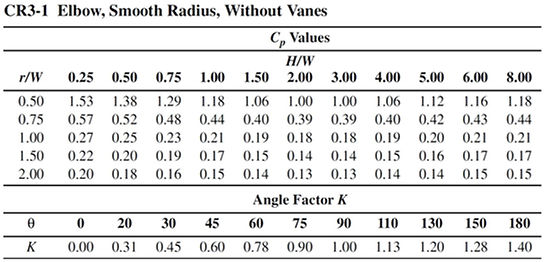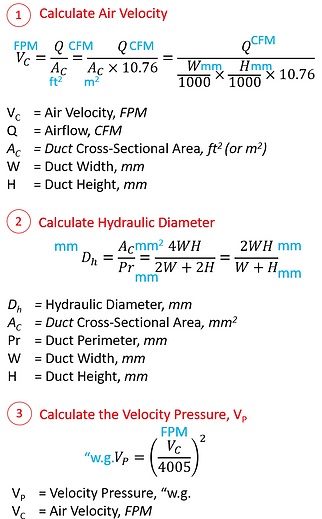top of page

Pressure Drop Calculation for Smooth-Radius Elbow

This calculator roughly estimates the pressure drop of smooth rectangular elbow (CR3-1) with reference to Ashrae Handbook Fundamentals.The formula for the computation of pressure drop, ΔP = KCpVp. The steps to compute ΔP are elaborated below.While the simple illustration above shows an example of how to determine the coefficient of loss, Cp with H/W and r/W from the Ashrae Table, the illustrations below show a few example of how r/W can be determined.Attached below a downloadable Excel File, and an Online Calculator for the calculation of a smooth elbow's pressure drop

Smooth Elbow Pressure Drop Calculator

bottom of page